### IMO Shortlist 2010 problem G4

Kvaliteta:
Avg: 3,0
Težina:
Avg: 7,0
Given a triangle$ABC$, with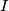$I$ as its incenter and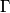$\Gamma$ as its circumcircle,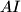$AI$ intersects$\Gamma$ again at$D$. Let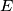$E$ be a point on the arc$BDC$, and$F$ a point on the segment$BC$, such that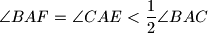$\angle BAF=\angle CAE < \dfrac12\angle BAC$. If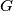$G$ is the midpoint of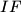$IF$, prove that the meeting point of the lines$EI$ and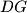$DG$ lies on$\Gamma$.

Proposed by Tai Wai Ming and Wang Chongli, Hong Kong
Izvor: Međunarodna matematička olimpijada, shortlist 2010